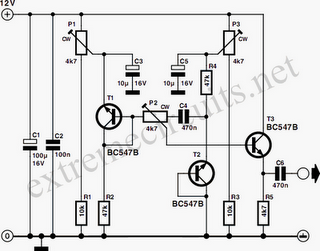# Learning Electronics

Learn to build electronic circuits

# Symmetric Noise Source

If a transistor junction operating in Zener breakdown is used as a noise source, the amplitude of the noise signal is asymmetric. This problem can be solved by using two transistors as two independent noise sources. One of these has a series resistor to earth, and the other has a series resistor to the supply line. Each of these noise sources produces an asymmetric noise voltage, with opposite asymmetry. If these two voltages are combined, the amplitude of the result will be symmetric. In the circuit diagram, T1 and T2 are the noise sources. The series resistors are R2 (to earth) and R4 (to the positive supply line).

The supply voltage for the noise sources has been made adjustable, to allow the noise generation of the transistors to be optimized. This is because the amount of noise produced depends on the power supply voltage. P1 and R1 provide an adjustable supply voltage between 8 and 12 V for the noise stage around T1, while P3 and R3 perform the same function for T2. C3 and C5 smooth these voltages. Since the amplitudes of the two noise sources will never be the same, it is necessary to take a weighted sum of the two signals. Consequently, P2 is included between the outputs of the noise sources as a sort of balance control.Since the DC levels of the two noise sources are not the same, C4 is also included in the balance network. The weighted sum of the two signals is present on the wiper of P2, superimposed on the DC signal of noise source T1. This DC level is also used for the DC bias of the buffer stage T3. The buffer isolates the noise sources from whatever circuit is connected to the output. To adjust the circuit, connect an oscilloscope to the output. First, turn P2 all the way to the left. Now rotate P1 until a maximum noise signal is seen on the oscilloscope. Next, turn P2 all the way to the right, and then adjust P3 for the best noise signal. Finally, adjust P3 so that the noise signal looks symmetric. The circuit provides an output voltage of approximately 150mV pp. The current consumption is 2mA. The oscilloscope shows the asymmetric noise signal on channel 2, and the symmetric noise signal on channel 1.